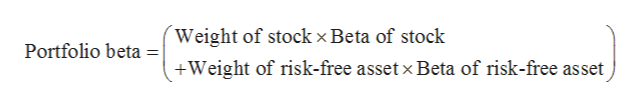# A stock has a beta of 1.8 and an expected return of 13 percent. A risk-free asset currently earns 3.2 percent.  If a portfolio of the two assets has a beta of 3.6, what are the portfolio weights? (Do not round intermediate calculations. A negative answer should be indicated by a minus sign. Round your answers to the nearest whole number, e.g., 32.)

Question
27 views

A stock has a beta of 1.8 and an expected return of 13 percent. A risk-free asset currently earns 3.2 percent.  If a portfolio of the two assets has a beta of 3.6, what are the portfolio weights? (Do not round intermediate calculations. A negative answer should be indicated by a minus sign. Round your answers to the nearest whole number, e.g., 32.)

check_circle

Step 1

Assume that the weight of risk-free asset be y. Thus, the weight of stock be (1-y).

The below expression can be used to calculate the weight of stock.help_outlineImage TranscriptioncloseWeight of stock x Beta of stock Portfolio beta Weight of risk-free asset x Beta of risk-free asset fullscreen
Step 2

Substitute (1-y) for weight of stock, 1.8 for beta of stock, y for weight of risk-free asset, 0 for beta of risk-free asset and 3.6 for portfolio beta,

Step 3

Weight for risk-free asset is -1. Thus, weight ...

### Want to see the full answer?

See Solution

#### Want to see this answer and more?

Solutions are written by subject experts who are available 24/7. Questions are typically answered within 1 hour.*

See Solution
*Response times may vary by subject and question.
Tagged in